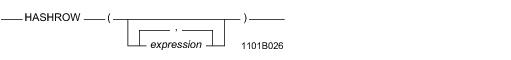# 15.00 - HASHROW - Teradata Database

## Teradata Database SQL Functions, Operators, Expressions, and Predicates

Product
Release Number
15.00
Content Type
Programming Reference
Publication ID
B035-1145-015K
Language
English (United States)

## HASHROW

### Purpose

Returns the hexadecimal row hash value for an expression or sequence of expressions. If no expression is specified, HASHROW returns the maximum hash code value.

### Syntaxwhere:

 Syntax element … Specifies … expression an optional expression or comma-separated list of expressions that can appear in the expression list of the select clause of a SELECT statement; typically a comma-separated list of column names that make up a (potential) index. HASHROW does not support expressions that result in UDT data types.

### ANSI Compliance

This is a Teradata extension to the ANSI SQL:2011 standard.

### Result

The resulting row hash value is typed BYTE(4).

 IF the argument list is … THEN HASHROW … empty returns the maximum hash code value. an expression that evaluates to NULL returns '00000000'XB. a list of expressions where all the expressions evaluate to NULL an expression that evaluates to 0, '', ' ', or a similar value a valid, non-NULL expression that can appear in the select list of a SELECT statement evaluates expression or the list of expressions and applies the hash function on the result. HASHROW returns the resulting row hash value. a list of expressions that can appear in the select list of a SELECT statement, where some expressions can evaluate to NULL

### Usage Notes

HASHROW is particularly useful for identifying the statistical properties of the current primary index, or to evaluate these properties for other columns to determine their suitability as a future primary index. You can also use these statistics to help minimize hash synonyms and enhance the uniformity of data distribution.

There are a maximum of 4,294,967,295 hash codes available in the system, ranging from '00000000'XB to 'FFFFFFFF'XB.

You can embed a HASHROW call within a HASHBUCKET call. For information on HASHBUCKET, see “HASHBUCKET” on page 898.

### Example

If you call HASHROW without an argument, it returns 'FFFFFFFF'XB, which is the maximum hash code in the system.

`   SELECT HASHROW(); `

### Example

The following example returns the average number of rows per row hash, where columns date_field and time_field constitute the primary index of the table eventlog.

`   SELECT COUNT(*) / COUNT(DISTINCT HASHROW (date_field,time_field)) `
`   FROM eventlog;`

If columns date_field and time_field qualify for a unique index, this example returns the average number of rows with the same hash synonym.

### Example

The following example evaluates the efficiency of changing the decimal format of a numeric field to eliminate synonyms.

Assume that column_1 and column_2 are declared as DECIMAL(2,2).

You can determine the effect of reformatting the columns to DECIMAL(8,6) and DECIMAL(8,4) on hash collisions by submitting these two queries.

`   SELECT COUNT (DISTINCT column_1(DECIMAL(8,6)) ||`
`   column_2(DECIMAL(8,4))`
`   FROM T;`
`   `
`   SELECT COUNT (DISTINCT HASHROW (column_1(DECIMAL(8,6)),    column_2 (DECIMAL(8,4)))`
`   FROM T;`

If the result of the second query is significantly less than the result of the first query, there are a significant number of hash collisions. That is, the closer the second result is to the first value indicates elimination of more hash synonyms.